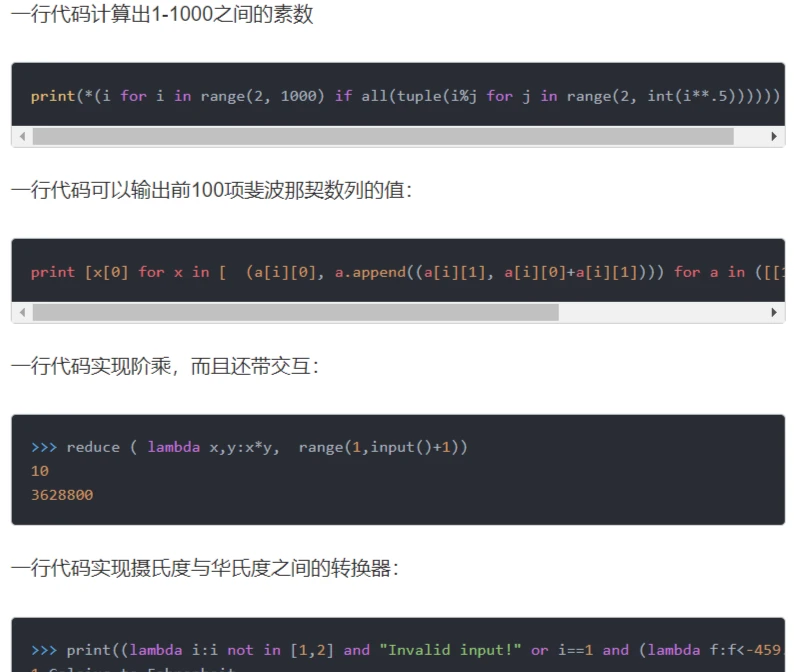# 背景

``fact(double n) => n < 0 ? throw ('n must be bigger than 0') : n == 0 ? 1 : n * fact(n - 1);``

# 解法

``fact = lambda x : 1 if x == 1 or x == 0 else x * fact(x - 1)``

``fact = lambda x : 1 if x == 1 or x == 0 else (x * fact(x - 1) if isinstance(x, int) and x > 0 else (_ for _ in ()).throw(ValueError('Illegal parameter')))``

# 附（顺便说一句，我之前查我的代码有没有先例，看到了这个，不过不符合要求）

目录